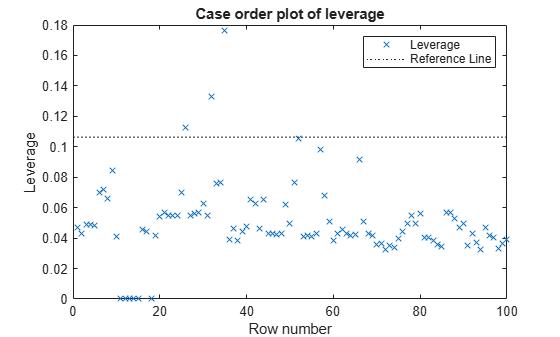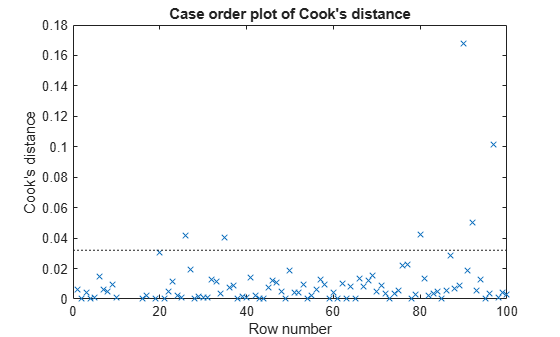# plotDiagnostics

Plot observation diagnostics of linear regression model

## Syntax

``plotDiagnostics(mdl)``
``plotDiagnostics(mdl,plottype)``
``plotDiagnostics(mdl,plottype,Name,Value)``
``h = plotDiagnostics(___)``

## Description

`plotDiagnostics` creates a plot of observation diagnostics such as leverage, Cook's distance, and delete-1 statistics to identify outliers and influential observations.

example

````plotDiagnostics(mdl)` creates a leverage plot of the linear regression model (`mdl`) observations. A dotted line in the plot represents the recommended threshold values.```
````plotDiagnostics(mdl,plottype)` specifies the type of observation diagnostics `plottype`.```
````plotDiagnostics(mdl,plottype,Name,Value)` specifies the graphical properties of diagnostic data points using one or more name-value pair arguments. For example, you can specify the marker symbol and size for the data points.```
````h = plotDiagnostics(___)` returns graphics objects for the lines or contour in the plot using any of the input argument combination in the previous syntaxes. Use `h` to modify the properties of a specific line or contour after you create the plot. For a list of properties, see Line Properties and Contour Properties.```

## Examples

collapse all

Plot the leverage values and Cook's distances of observations and find the outliers.

Load the `carsmall` data set and fit a linear regression model of the mileage as a function of model year, weight, and weight squared.

```load carsmall tbl = table(MPG,Weight); tbl.Year = categorical(Model_Year); mdl = fitlm(tbl,'MPG ~ Year + Weight^2');```

Plot the leverage values.

```plotDiagnostics(mdl) legend('show') % Show the legend```The dotted line represents the recommended threshold value 2*p/n, where p is the number of coefficients, and n is the number of observations. Find the threshold value using the `NumCoefficients` and `NumObservations` properties.

`t_leverage = 2*mdl.NumCoefficients/mdl.NumObservations`
```t_leverage = 0.1064 ```

Find the observations with leverage values that exceed the threshold value.

`find(mdl.Diagnostics.Leverage > t_leverage)`
```ans = 3×1 26 32 35 ```

You can also find an observation number by using a data tip. Select the data points above the threshold line to display their data tips. The data tip includes the x-axis and y-axis values for the selected point, along with the observation number.

Plot the Cook's distance values.

`plotDiagnostics(mdl,'cookd')`The dotted line represents the recommended threshold value. Compute the threshold value `t_cookd`.

`t_cookd = 3*mean(mdl.Diagnostics.CooksDistance,'omitnan')`
```t_cookd = 0.0320 ```

Find the observations with the Cook's distance values that exceed the threshold value.

`find(mdl.Diagnostics.CooksDistance > t_cookd)`
```ans = 6×1 26 35 80 90 92 97 ```

Two observations (26 and 35) are outliers by both measures, but some points (32, 80, 90, 92, and 97) are outliers by only one measure.

## Input Arguments

collapse all

Linear regression model, specified as a `LinearModel` object created using `fitlm` or `stepwiselm`.

Type of plot, specified as one of the values in this table.

ValuePlot TypeDotted Reference Line in Plot Purpose
`'contour'`Residual vs. leverage with overlaid contours of Cook's distanceContours of Cook's distanceIdentify observations with large residual values, high leverage, and large Cook's distance values.
`'cookd'`Cook's distanceRecommended threshold, computed by `3*mean(mdl.Diagnostics.CooksDistance)`Identify observations with large Cook's distance values.
`'covratio'`Delete-1 ratio of determinant of covarianceRecommended thresholds, computed by `1±3*p/n`, where `p` is the number of coefficients (`mdl.NumCoefficients`) and `n` is the number of observations (`mdl.NumObservations`)Identify observations where the delete-1 statistic value is not in the range of the recommended thresholds.
`'dfbetas'`Delete-1 scaled differences in coefficient estimatesRecommended threshold, computed by `3/sqrt(n)`Identify observations with large delete-1 statistic values.
`'dffits'`Delete-1 scaled differences in fitted valuesRecommended threshold, computed by `2*sqrt(p/n)` in an absolute valueIdentify observations with large delete-1 statistic values in an absolute value.
`'leverage'`LeverageRecommended threshold, computed by `2*p/n`Identify high leverage observations.
`'s2_i'`Delete-1 varianceMean squared error (`mdl.MSE`)Compare the delete-1 variance with the mean squared error.

For all plot types except `'contour'`, the x-axis is the row number (case order) of observations.

The `Diagnostics` property of `mdl` contains the diagnostic values used by `plotDiagnostics` to create plots.

### Name-Value Arguments

Specify optional pairs of arguments as `Name1=Value1,...,NameN=ValueN`, where `Name` is the argument name and `Value` is the corresponding value. Name-value arguments must appear after other arguments, but the order of the pairs does not matter.

Before R2021a, use commas to separate each name and value, and enclose `Name` in quotes.

Example: `'Color','blue','Marker','o'`

Note

The graphical properties listed here are only a subset. For a complete list, see Line Properties. The specified properties determine the appearance of diagnostic data points.

Line color, specified as the comma-separated pair consisting of `'Color'` and an RGB triplet, hexadecimal color code, color name, or short name for one of the color options listed in the following table.

The `'Color'` name-value pair argument also determines marker outline color and marker fill color if `'MarkerEdgeColor'` is `'auto'` (default) and `'MarkerFaceColor'` is `'auto'`.

For a custom color, specify an RGB triplet or a hexadecimal color code.

• An RGB triplet is a three-element row vector whose elements specify the intensities of the red, green, and blue components of the color. The intensities must be in the range `[0,1]`, for example, ```[0.4 0.6 0.7]```.

• A hexadecimal color code is a string scalar or character vector that starts with a hash symbol (`#`) followed by three or six hexadecimal digits, which can range from `0` to `F`. The values are not case sensitive. Therefore, the color codes `"#FF8800"`, `"#ff8800"`, `"#F80"`, and `"#f80"` are equivalent.

Alternatively, you can specify some common colors by name. This table lists the named color options, the equivalent RGB triplets, and hexadecimal color codes.

Color NameShort NameRGB TripletHexadecimal Color CodeAppearance
`"red"``"r"``[1 0 0]``"#FF0000"``"green"``"g"``[0 1 0]``"#00FF00"``"blue"``"b"``[0 0 1]``"#0000FF"``"cyan"` `"c"``[0 1 1]``"#00FFFF"``"magenta"``"m"``[1 0 1]``"#FF00FF"``"yellow"``"y"``[1 1 0]``"#FFFF00"``"black"``"k"``[0 0 0]``"#000000"``"white"``"w"``[1 1 1]``"#FFFFFF"``"none"`Not applicableNot applicableNot applicableNo color

Here are the RGB triplets and hexadecimal color codes for the default colors MATLAB® uses in many types of plots.

`[0 0.4470 0.7410]``"#0072BD"``[0.8500 0.3250 0.0980]``"#D95319"``[0.9290 0.6940 0.1250]``"#EDB120"``[0.4940 0.1840 0.5560]``"#7E2F8E"``[0.4660 0.6740 0.1880]``"#77AC30"``[0.3010 0.7450 0.9330]``"#4DBEEE"``[0.6350 0.0780 0.1840]``"#A2142F"`Example: `'Color','blue'`

Line width, specified as the comma-separated pair consisting of `'LineWidth'` and a positive value in points. If the line has markers, then the line width also affects the marker edges.

Example: `'LineWidth',0.75`

Marker symbol, specified as the comma-separated pair consisting of `'Marker'` and one of the values in this table.

MarkerDescriptionResulting Marker
`"o"`Circle`"+"`Plus sign`"*"`Asterisk`"."`Point`"x"`Cross`"_"`Horizontal line`"|"`Vertical line`"square"`Square`"diamond"`Diamond`"^"`Upward-pointing triangle`"v"`Downward-pointing triangle`">"`Right-pointing triangle`"<"`Left-pointing triangle`"pentagram"`Pentagram`"hexagram"`Hexagram`"none"`No markersNot applicable

Example: `'Marker','+'`

Marker outline color, specified as the comma-separated pair consisting of `'MarkerEdgeColor'` and an RGB triplet, hexadecimal color code, color name, or short name for one of the color options listed in the `Color` name-value pair argument.

The default value of `'auto'` uses the same color specified by using `'Color'`.

Example: `'MarkerEdgeColor','blue'`

Marker fill color, specified as the comma-separated pair consisting of `'MarkerFaceColor'` and an RGB triplet, hexadecimal color code, color name, or short name for one of the color options listed in the `Color` name-value pair argument.

The `'auto'` value uses the same color specified by using `'Color'`.

Example: `'MarkerFaceColor','blue'`

Marker size, specified as the comma-separated pair consisting of `'MarkerSize'` and a positive value in points.

Example: `'MarkerSize',2`

## Output Arguments

collapse all

Graphics objects corresponding to the lines or contour in the plot, returned as a graphics array. Use dot notation to query and set properties of the graphics objects. For details, see Line Properties and Contour Properties.

You can use name-value pair arguments to specify the appearance of diagnostic data points corresponding to the first graphics object `h(1)`. If `plottype` is `'dfbetas'`, the plot includes a line object for each coefficient. Name-value pair arguments specify the line object properties of all coefficients. You can modify the properties of each coefficient separately by using the corresponding graphics object.

collapse all

### Cook’s Distance

Cook’s distance is the scaled change in fitted values, which is useful for identifying outliers in the X values (observations for predictor variables). Cook’s distance shows the influence of each observation on the fitted response values. An observation with Cook’s distance larger than three times the mean Cook’s distance might be an outlier.

Each element in the Cook's distance D is the normalized change in the fitted response values due to the deletion of an observation. The Cook’s distance of observation i is

`${D}_{i}=\frac{\sum _{j=1}^{n}{\left({\stackrel{^}{y}}_{j}-{\stackrel{^}{y}}_{j\left(i\right)}\right)}^{2}}{p\text{\hspace{0.17em}}MSE},$`

where

• ${\stackrel{^}{y}}_{j}$ is the jth fitted response value.

• ${\stackrel{^}{y}}_{j\left(i\right)}$ is the jth fitted response value, where the fit does not include observation i.

• MSE is the mean squared error.

• p is the number of coefficients in the regression model.

Cook’s distance is algebraically equivalent to the following expression:

`${D}_{i}=\frac{{r}_{i}^{2}}{p\text{\hspace{0.17em}}MSE}\left(\frac{{h}_{ii}}{{\left(1-{h}_{ii}\right)}^{2}}\right),$`

where ri is the ith residual, and hii is the ith leverage value.

For more details, see Cook’s Distance.

### Delete-1 Statistics

Delete-1 statistics are useful for finding the influence of each observation. These statistics capture the changes that would result from excluding each observation in turn from the fit. If the delete-1 statistics differ significantly from the model using all observations, then the observation is influential.

See Delete-1 Statistics for the definitions and usages of the delete-1 statistics.

### Leverage

Leverage is a measure of the effect of a particular observation on the regression predictions due to the position of that observation in the space of the inputs.

The leverage of observation i is the value of the ith diagonal term hii of the hat matrix H. The hat matrix H is defined in terms of the data matrix X:

H = X(XTX)–1XT.

The hat matrix is also known as the projection matrix because it projects the vector of observations y onto the vector of predictions $\stackrel{^}{y}$, thus putting the "hat" on y.

Because the sum of the leverage values is p (the number of coefficients in the regression model), an observation i can be considered an outlier if its leverage substantially exceeds p/n, where n is the number of observations.

For more details, see Hat Matrix and Leverage.

## Tips

• The data cursor displays the values of the selected plot point in a data tip (small text box located next to the data point). The data tip includes the x-axis and y-axis values for the selected point, along with the observation name or number.

• Use `legend('show')` to show the pre-populated legend.

## Alternative Functionality

 Neter, J., M. H. Kutner, C. J. Nachtsheim, and W. Wasserman. Applied Linear Statistical Models, Fourth Edition. Chicago: McGraw-Hill Irwin, 1996.